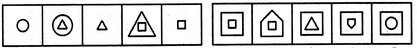# Non Verbal Reasoning - Series - Discussion

Discussion Forum : Series - Section 1 (Q.No. 11)
Directions to Solve

Each of the following questions consists of five figures marked A, B, C, D and E called the Problem Figures followed by five other figures marked 1, 2, 3, 4 and 5 called the Answer Figures. Select a figure from amongst the Answer Figures which will continue the same series as established by the five Problem Figures.

11.

Select a figure from amongst the Answer Figures which will continue the same series as established by the five Problem Figures.(A)     (B)     (C)     (D)     (E)       (1)     (2)     (3)     (4)     (5)
1
2
3
4
5
Explanation:
In one step, the existing element enlarges and a new element appears inside this element. In the next step, the outer element is lost.
Discussion:
11 comments Page 1 of 2.

Prakash Kadam said:   2 years ago
Correct Answer, Thanks @Parthipan & Jack.

Hapil said:   2 years ago
Ans: D (4)

Explanation:
Figure 1 has a circle, figure 2 shows a triangle inside a circle and figure 3 shows a triangle

Therefore we could conclude that the circle shows the way to the next pattern which is a triangle

we could come to an option that the answer has an outer square
- None of the shapes in the questions should be included in the answer

Answer (1) has q square inside the outer square,so not possible since question already has a square.
Answer (2) does not have an outer square.
Answer (3) has a triangle inside the outer square, not possible since question already has a triangle.
Answer (4) it is correct, because it has a pentagon representing the next order, and it's not fond in the question and has an outer square.

Parthipan said:   5 years ago
It should be E only, because Triangle is having 3 sides which comprises of square (4 sides) in side it in Dth position of the question. Similarly, Square having 4 sides will be comprising of Pentagon (5 sides). So, answer is D only.

Prabh said:   5 years ago
Why it is not E?

Prabh said:   5 years ago
Why it is not E?

Ganesh said:   7 years ago
We should consider the inner figure also I feel. Option D has a pentagon but it is inverted. That's why I feel it should not be the best option to ahead with but E.

Also if we consider number of times a figure is repeated in the series then both triangle and square are repeated 3 times but the circle only two times. So E is best suitable option in this case.

Bikash said:   8 years ago
D is the correct answer because the number of sides of the inner shapes are increasing by +1 accordingly.

Ron said:   9 years ago
But if it is seen as a circular loop then E might be the write answer, Otherwise D is fine. @Jack's logic is reasonable.

First you have a triangle (3 sides), then a square (4 sides) so the next figure should be a pentagon (5 sides).